Home    |    Teacher    |    Parents    |    Glossary    |    About UsIf two objects have the same shape, they are called "similar." When two figures are similar, the ratios of the lengths of their corresponding sides are equal. To determine if the triangles shown are similar, compare their corresponding sides. Are these ratios equal?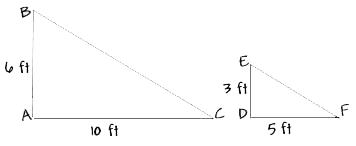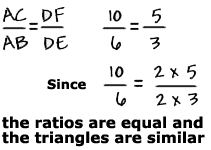Triangle ABC is similar to triangle DEF. We can write this using a special symbol, as shown here.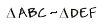Side AB corresponds to Side DE, Side AC corresponds to Side DF, and Side BC corresponds to side EF.

If you know that two objects are similar, you can use proportions and cross products to find the length of an unknown side. Let's find the length of side DF, labeled x.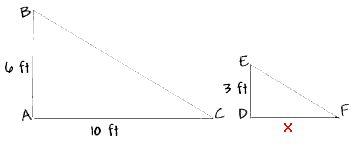We can write a proportion, like this: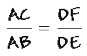We read this proportion as: "AC is to AB as DF is to DE." Now, substitute in the lengths of the sides.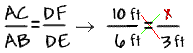Take the cross product to get the equation.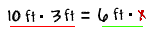Solve the equation.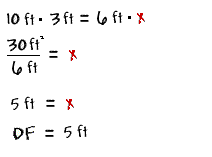Homework Help | Pre-Algebra | Ratios and ProportionsEmail this page to a friendSearch·  Ratios·  Proportions·  Distance, rate     and time·  Similar figuresFirst Glance In Depth Examples WorkoutSimilar figures